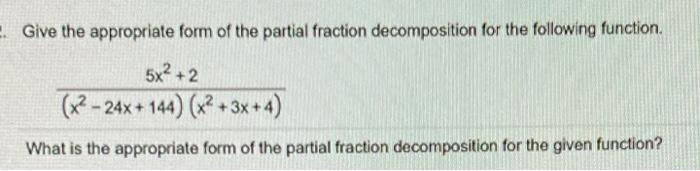# Question Solve it with complete steps ! I have post the answer also. . Give the appropriate form of the partial fraction decomposition for the following function. 5x2 + 2 (x2 - 24x + 144) (x2 +3x+4) What is the appropriate form of the partial fraction decomposition for the given function? Answer: A B Cx+D + X - 12 (x - 122x2 + 3x + 4 +